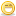1. ##Desired Airflow Question, not your normal question

What sensor(s) does the ecm read to determine how much airflow you need?

Maf? but what about when its in map only mode?Reply With Quote

2. ##Originally Posted by Dale
What sensor(s) does the ecm read to determine how much airflow you need?

Maf? but what about when its in map only mode?
I think you will find if its not refierencing the MAF then its ONLY looking up the Main VE table B0101 or B0200 (Speed Density)Reply With Quote

3. ##The PCM doesn't know how much air flow an engine needs, you have to tell it. That's the purpose of the "Desired Air Flow" table. As for the measurement to determine actual air flow, that's typically done by Mass Air Flow sensor readings. Of course, that brings up the question of how air flow is determned in speed density systems. The PCM accomplishes that by computation using engine displacement, engine speed and MAP sensor readings.Reply With Quote

4. ##Originally Posted by dfe1
The PCM accomplishes that by computation using engine displacement, engine speed and MAP sensor readings.
Ok, its using the RPM and MAP sensors, and the main VE table.Reply With Quote

5. ##Ok, thanks. So if I have the incorrect maf sensor, then its f-ing everything up.

Ok, time to go get the propper maf and force it to fit.Reply With Quote

6. ##Calculating cylinder airmass (open loop)

The VE table's "real" units are g*K/kPa

where:
g is grams (mass)
K is degrees Kelvin (temperature)
kPa is kilopascals (pressure)

so each VE table cell value is the "normalized" cylinder airmass...
i.e. "normalized" for absolute termperature and absolute pressure;

as temperature goes down, airmass goes up (i.e. mass varies "per" 1/K),
as pressure goes up, airmass goes up (i.e. mass varies "per" kPa),
so the units are: g /(1/K) /kPa == g/(kPa/K) == g*K/kPa.

to calculate the cylinder airmass, the PCM reads the VE table cell value and divides by the absolute temperature and multiplies by the absolute pressure;

e.g.
very simplistically, say:
- the PCM computues (via IAT/ECT temperature blending) the air temp to be 300K (27°C, 80°F),
- VE table says 2.0 g*K/kPa,
- MAP is 100 kPa,
then airmass = 2.0[g*K/kPa] / 300[K] * 100[kPa] = 0.6667[g]

(note how the units balance out)Reply With Quote

7. ##Continuing the example...

Then say the commanded AFR is 13.3, so
fuelmass = airmass / AFR = 0.6667[g] / 13.3 = 0.0501[g]

say the IFR is 4.175 g/s, then
injector pulse width (IPW) = fuelmass / IFR = 0.0501[g] / 4.175[g/s] = 0.012[s] = 12.0[ms]

and [say] engine speed is 6000 RPM (100 rev/s),
for sequential injection, each injector fires once every 2 revs (4 strokes), so
4 stroke period = 2[rev] / 100[rev/s] = 0.02[s] = 20.0[ms]
so injector duty cycle (IDC) = IPW / period * 100% = 12.0[ms] / 20.0[ms] * 100% = 60.0%.

The IDC is the end result of obtaining the airmass from the VE table,
and computing the fuelmass needed for the commanded AFR,
and computing how long to open the injectors to spray that amount of fuel.

Just so you see the "big" picture...Reply With Quote

8. ##Originally Posted by Gelf VXR
Ok, its using the RPM and MAP sensors, and the main VE table.
No, if I understand your original question correctly, the VE table is not a consideration. When you asked about the amount of air flow you need, I assumed your question was in reference to the "Desired Air Flow" table (B4307). If that's the case, that table has to do with the amount of air required for idle. The entries in this table typically have to be adjusted when a long duration camshaft is installed or any other modification that significantly alters idle air flow characteristics is done.Reply With Quote

9. ##Originally Posted by Dale
What sensor(s) does the ecm read to determine how much airflow you need?

Maf? but what about when its in map only mode?
I believe that is uses RPM in either maf or SD mode along with tables
B4512
B4514
B4515
B4521
I am happy to be corrected though as I have only just started looking into these tables.Reply With Quote

10. ##4521 limits how much the pcm will correct. Its part of what I'm dealing with, but not the fully what I was looking for in the original post.

The others I dont understand.Reply With Quote

####Posting Permissions

• You may not post new threads
• You may not post replies
• You may not post attachments
• You may not edit your posts
•